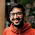## Wednesday, 18 July 2012

### Selection through identical balls

You randomly withdraw 3 balls out of a lot containing identical red, identical green and identical blue balls. What is the probability that you get 1 red, 1 green and 1 blue ball given the number of
a) Red balls = 3,  Green balls = 3,  Blue balls = 3
b) Red balls = p,  Green balls = q,  Blue balls = r such that p,q,r>3
c) Red balls = infinity,  Green balls = infinity,  Blue balls = infinity

#### 6 comments:

1.the red can be chosen p ways, for each of the way green can be chosen q ways and for each of the previous selection blue can be chosen r ways... so total no of ways one can chose 1 red, 1 green & 1 blue is = p*q*r ..... and total no of ways one can chose 3 balls from (p+q+r) is (p+q+r)C3 ... so probability is p*q*r/(p+q+r)C3 ...

now for each ball of infinite quantity, assume the infinity is of same category
(there are different categories of infinity.. as set of natural no and set of real no both are infinite but of different kind) ... so put p=q=r=n, we get prob. = n^3/3nC3 ... and let n-> infinity, we get prob = 2/9 ...

we can get the ans in a different way... first pick up a ball (it will be of one of the three color), then the next ball you pick will be of different color with 2/3 prob.... then chose the remaining color (we have already two different color ball in hand) with prob. 1/3 ... so total prob. is = 2/3 * 1/3 = 2/9 ...

2.This comment has been removed by the author.

3.Number of ways of selecting ‘r’ things from ‘n’ identical things is ‘1’.

Therefore, first answer would be 1.1.1/(number of solutions of x+y+z=3, where x,y,z>=0) i.e. 1/10

For second part : 1/10, since p,q,r > 3

For third part : 1/10. Same logic.

4.@sumit, here we have RRR GGG BBB balls, and you consider RGB choice as only '1' way of picking it, but choosing R1G1B1 is not same as R1G1B2, as we are actually picking the balls from the urn and the true essence of 'identical' things is being violated....

by the way i get my answer in another way... suppose we first choose a color R in 3/9 ways, then we choose G in 3/8 ways (since only 8 balls remaining) and then choose B in 3/7 ways... we do the seq. RGB or RBG or GRB or GBR or BRG or BGR - total 6 ways... so prob is 3/9 * 3/8 * 3/7 * 6 = 6/56, as obtained before (n^3/3nC3 = 3^3/9C3 = 6/56)..

@sumit, can you please tell me the mistake of the argument I gave?

5.the balls are identical but distinguishable... so @sumit, what you have calculated is for indistinguishable balls...

6.@rik sorry but the balls are identical and indistinguishable
@sumit I still doubt the exact answer but look at this

What is the probability to select a red ball out of a lot of 1 red ball and infinite blue balls?
As per your logic - x1+x2 = 1
Answer should be 1/2 = 0.5
But should it be 0.5 or should it be 0